## Introduction

A spin liquid can be considered as a Mott insulator that is not adiabatically connected to any band insulator and does not break any symmetry even at zero temperature. Recently, much attention has been focused on a possible spin liquid in two or three spatial dimensions1,2,3,4,5. On one hand, it has been suggested experimentally that several organic materials represent good candidates for spin liquids6,7,8. On the other hand, the existence of a spin liquid has so far been demonstrated only in very few and particularly simplified models9,10.

In order to understand this issue, let us consider a model Hamiltonian on a lattice describing the insulating state of electrons at half-filling, i.e., one electron per lattice site. Since the charge gap is assumed, only spin degrees of freedom remain and can be described by the spin-1/2 Heisenberg model. Since any spin-1/2 model corresponds to a well defined interacting hard core boson model, the crucial question is to understand how – at zero temperature – these bosonic degrees of freedom can avoid Bose-Einstein condensation and/or crystallization, necessary conditions for a stable spin liquid with no long-range order of any kind.

In this report, we study the ground state of the half-filled Hubbard model on the honeycomb lattice (see Fig. 1) defined by the following Hamiltonian,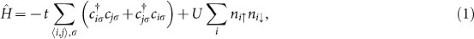where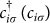is a creation (annihilation) operator of spin up/down (σ = ↑,↓) electrons on lattice site i,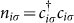and t (U) denotes the nearest-neighbor hopping (on-site repulsion). This is known to be a model Hamiltonian for graphene with U/t ≈ 311. More importantly, it is not geometrically frustrated, namely, as seen in Fig. 1, the neighboring sites of any site on A sublattice belong to B sublattice (and vice versa). Indeed, it is well known that the ground state becomes an antiferromagnetic insulator (AFMI), i.e., classically Néel ordered, from a semi-metal (SM) with increasing U/t12.

Very recently, Meng et al.2 has reexamined the ground state phase diagram of this model and found a possible spin liquid phase in the range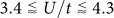between SM and AFMI. Their finding is rather surprising because it is widely believed that a stable spin liquid occurs most likely in frustrated quantum systems where strong quantum fluctuations destroy the long-range magnetic order even at zero temperature1. Their study was particularly successful because, with the auxiliary field technique13, there is no sign problem in the corresponding quantum Monte Carlo simulations and an accurate finite size scaling was possible by using numerically exact results for clusters containing up to 648 sites. So far, their results represent the most important numerical evidence for a possible spin liquid ground state in a “realistic” electronic model in two dimensions (2D), because, to our knowledge, only a particularly simplified quantum dimer model on the triangular lattice9 and the Kitaev model10, built ad hoc to have an exact solution, allow a spin liquid ground state in 2D. Furthermore, their results were considered to be a clear violation of the “Murphy's Law”: in a too simple model, not vexed by the “fermion sign problem”, nothing interesting can occur5.

Here, by performing simulations for much larger clusters containing up to 2592 sites, we show that antiferromagnetic order concomitantly occurs once the insulating behavior sets in, supporting the more conventional Hartree-Fock (HF) transition from SM to AFMI12. Although our results agree with the previous study for the same clusters up to 648 sites, we have reached a quite different conclusion, as the possible spin liquid region reduces substantially to a small interval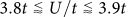, if it ever exists. This reminds us similar claims on spin liquid behaviors in different systems in 2D14,15, which have been corrected later on by much larger cluster simulations, showing instead antiferromagnetic long-range order16,17.

## Results

We use finite size clusters of N = 2L2 sites (thus containing L × L unit cells) with periodic boundary conditions (see Fig. 1), which satisfy all symmetries of the infinite lattice18 (also see Supplementary information). Here L is the linear dimension of the cluster and we take L up to 36. We use the well established auxiliary field Monte Carlo technique13, which allows the statistical evaluation of the following quantity,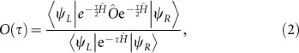where Ô is a physical operator, |ψR〉 (|ψL〉) is the right (left) trial wave function (not orthogonal to the exact ground state) and τ is the projection time. The exact ground state expectation value 〈Ô〉 of the operator Ô is then obtained by adopting the limit of τ → ∞ and Δτ → 0 for O(τ), where Δτ is the short time discretization of τ. This approximation – the so called Trotter approximation – is necessary to introduce the auxiliary fields13 and implies a systematic error, negligible for small Δτ (see Supplementary information).

First, we study both the spin structure factor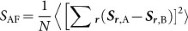and the spin-spin correlations Cs(R) = 〈Sr,A·Sr+R,A〉 at the maximum distance |R| = Lmax of each cluster for U/t = 4, where the strongest evidence of a spin liquid behavior was found in Ref. 2. Here Sr,A (Sr,B) is the spin operator at unit cell r on A (B) sublattice. As shown in Fig. 2b, our results show consistently a finite value of the antiferromagnetic order parameter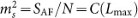for L → ∞, in sharp contrast to the existence of a spin liquid, i.e., spin disordered, ground state reported in Ref. 2.

By doing similar calculations for several U/t values (see Fig. 2 and Supplementary information), we find in Fig. 3 that ms approximately scales linearly with respect to U/t, i.e., ms |UUc|β, with a critical exponent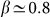, which is similar to the critical behavior (β = 1) predicted by the HF theory12. Although corrections to this almost linear critical behavior are obviously expected, they do not change much the critical value Uc at which the antiferromagnetic order melts, as clearly shown in Fig. 3. Our best estimate of the critical value is Uc/t = 3.869± 0.013, which is much smaller than the one (≈ 4.3) reported in Ref. 2. Note, however, that the critical exponent β may be different from the present estimate if the critical region is very close to Uc. In such case the accurate determination of β obviously requires much larger clusters which are not feasible at present.

Let us now evaluate the spin gap Δs. In order to avoid possible errors in extrapolating the imaginary time displaced spin-spin correlation functions, here we calculate directly the total energies in the singlet and the triplet sectors, with improved estimators, which dramatically reduce their statistical errors20 (also see Supplementary information). We can see clearly in Fig. 4a that the extrapolated spin gaps for different U/t values are always zero within statistical errors (e.g., the statistical error as small as 0.004t for U/t = 4).

Next, we investigate whether the system is metallic or insulating, namely, whether there exists a zero or a finite charge gap. For this purpose, it is enough to simply study the long distance behavior of charge-charge correlations,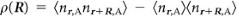. Here nr,A is the density operator at unit cell r on A sublattice (see Fig 1). They should change from power law to exponential behavior at a critical U where the charge gap opens up. This change of behavior is evident in Fig. 4b and appears consistently around the onset of the antiferromagnetic transition (Uc), within a remarkably small uncertainty < 0.1t on the value of U. Our results therefore strongly support the more conventional scenario of a direct and continuous quantum phase transition between SM and AFMI12.

## Discussion

Let us now discuss here why we have not found any evidence of a spin liquid phase. As shown in Ref. 21, by applying one of the theorems by Lieb22, it is easily proved that the exact ground state of this model for U ≠ 0 satisfies the Marshall sign rule23 in the sector of no doubly occupied sites, accounting for low energy spin excitations. Indeed, the phases coincide with those of the simple antiferromagnetic Néel state ordered along the x-spin quantization axis,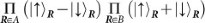, where |↑〉R and |↓〉R are spin configurations (along the z-spin quantization axis) at site R. The expansion of this state in terms of |↑〉R and |↓〉R yields the simple Marshall sign, namely, it is negative if the number of spin down in the A sublattice is odd. Thus, the phases of the ground state are trivial in the bosonic spin 1/2 sector. Therefore, Bose-Einstein condensation can hardly be avoided and a magnetic long-range order occurs once the charge gap becomes finite.

At this point, one could be tempted to assume the general validity of the above observation for generic S = 1/2 model Hamiltonians with SU(2) invariance and use this criteria based on the phases of the ground state wave function as a powerful guideline in the search of spin liquids for model systems as well as for real materials. Indeed, in all unfrustrated spin-1/2 Heisenberg and Hubbard models in the sector of no doubly occupied sites, the phases of the ground state wave function are not at all entangled in real space as they factorize into independent contributions relative to each site. Instead, the phases of the ground state wave functions are highly non trivial in well established spin liquid models such as, for instance, the Kitaev's model10 and the celebrated quantum dimer model on the triangular lattice9, because they are described by paired wave functions, which couple in a non trivial way the phases of nearest neighbor spins24.

Therefore, we conclude that in a true spin liquid in 2D, the phases of the ground state wave function should be highly non trivial and entangled, otherwise any seed of spin liquid behavior would be most likely destabilized. To our best knowledge, the above observation is valid so far for all spin-1/2 models with SU(2) invariance. Notice that the restriction to SU(2) invariant models appears to be important because the spin-1/2 easy-axis Heisenberg model on the Kagome lattice most likely display spin liquid behavior25,26,27. Here, however, the calculations have not been confirmed on fairly low temperatures yet. Therefore, further numerical study is required for understanding what are the key ingredients that stabilize a spin liquid phase in “realistic” electronic models.

## Methods

Here we employ the standard auxiliary field Monte Carlo algorithm13 with a more efficient implementation20 by using different left and right trial functions |ψL〉 and |ψR〉 in equation (2). We include also in the trial wave function a Gutzwiller type projection, exp(−gΣi nini), where g is the Gutzwiller variational parameter, to optimize the efficiency. As reported in Ref. 20, the statistical error in evaluating the energy E(S) for a given spin S is dramatically reduced for appropriate values of g. Thus we can evaluate the spin gap ΔS = E(S = 1) − E(S = 0) with high accuracy, without facing the negative sign problem, by directly simulating the singlet S = 0 and the triplet S = 1 sectors separately (see Supplementary information).

In order to accelerate the convergence to the ground state, we use for |ψR〉 a Slater determinant with a definite spin S, by breaking only spatial symmetries to remove the degeneracy at momenta K and K′ for the clusters chosen (a similar strategy was adopted in Ref. 2). Conversely, we use for |ψL〉 a rotational and translationally invariant Slater determinant obtained by diagonalizing a mean field Hamiltonian, containing an explicit antiferromagnetic order parameter directed along the x-spin quantization axis. In this way, the left and the right trial wave functions break different symmetries (spin and spatial ones, respectively) and for any symmetric operator Ô the convergence to the ground state is expected to be much faster because it is dominated by the singlet gap in the symmetric sector Δgap, that is clearly much larger than, e.g., the lowest triplet excitation in the magnetic phase. Since Δgap is expected to scale to zero (if indeed zero) at most as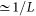, we use a projection time τ = (L + 4)/t, which we have tested carefully to be large enough for well converged results (see Supplementary information). We have also checked that the systematic error due to discretizing τ is basically negligible with Δτt = 0.14 for the spin gap calculations and with Δτt = 0.1 for the correlation functions (see Fig. 3 and Supplementary information).

In order to evaluate the statistical errors of the finite size extrapolations, we use a straightforward resampling technique. This resampling technique is used, for example, when values of SAF calculated for finite sizes are extrapolated to L → ∞ to estimate ms in Fig. 3. Let us denote in general the calculated Monte Carlo data f(L) and the corresponding statistical error δf(L) obtained for a cluster of size L. The main goal of this resampling technique is to estimate the finite-size extrapolated value c0 and its statistical error δc0 when the Monte Carlo data are fitted by, e.g., cubic polynomials,In this resampling technique, we first generate for each L a “fictitious sample” which is normally distributed around f(L) with its standard deviation δf(L), which is also an output of the quantum Monte Carlo simulation. Then, we fit these “fictitious” data to equation (3), by using the weighted (with 1/(δf(L))2) least square fit and estimate c0. We repeat this Mrs times so that we have now Mrs samples for c0, i.e.,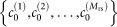distributed according to a probability distribution consistent with the Monte Carlo simulations. Finally, we simply average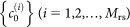for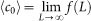and the standard deviation of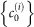gives an estimate of the statistical error δc0 of the extrapolated value 〈c0〉. We take Mrs = 200. We have checked that the resultant 〈c0〉 and δc0 are not dependent on Mrs as long as Mrs is large enough.